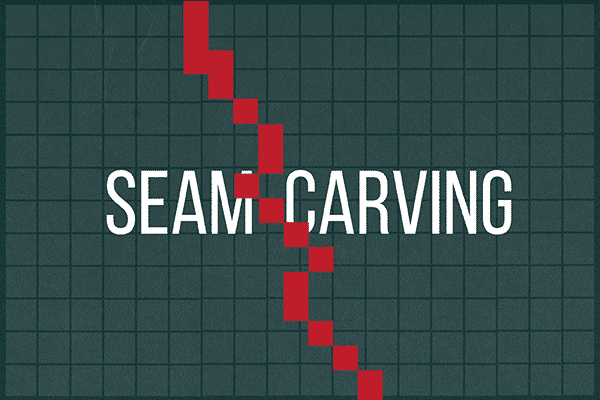## SeamCarving

2106 0 2017年11月20日

0 0 投票数

Seam Carving 算法与一般的图像裁剪技术不同，它可以保持图像最具有信息量的部分。

private int yielding(Color c1, Color c2) {
// 计算两个颜色的R、G、B差值
int r = (c1.getRed() - c2.getRed());
int g = (c1.getGreen() - c2.getGreen());
int b = (c1.getBlue() - c2.getBlue());
// 代入公式计算结果
return r * r + g * g + b * b;
}

// energy of pixel at column x and row y
public double energy(int x, int y) {
if (x < 0 || x >= width() || y < 0 || y >= height()) {
throw new IllegalArgumentException();
}

// 如果是边界情况，按照定义，能量是 1000
final int DEFAULT_ENERGY = 1000;
if (x == 0 || y == 0 || x == width() - 1 || y == height() - 1) {
return DEFAULT_ENERGY;
}

// 计算纵向的梯度
int yGradient = yielding(picture.get(x, y - 1), picture.get(x, y + 1));

// 计算横向的梯度
int xGradient = yielding(picture.get(x - 1, y), picture.get(x + 1, y));

}

1. 权重不是在边上，而是在顶点上。
2. 需要找一条最短路，从顶部的 W 个像素中的任意一个出发，到底部的 W 个像素中的任意一个。
3. 这条最短路是没有循环的，同时它是连续的，即 (x, y) 的下一个点只能是 (x + 1, y + 1), (x, y + 1) 和 (x – 1, y + 1) 中的任意一个。

// 遍历二维数组
for (int row = 0; row < picture.height(); ++row) {
for (int col = 0; col < picture.width(); ++col) {

if (row == 0) {
// 如果这是最上面的一行，那么最小能量花费显然就是它自己的能量
dynamicMinimumEnergy[row][col] = energy[row][col];
} else {
/*
* 如果不在最上面的一行，那么能量有三种路径可能到达 左上（如果存在）、正上、右上（如果存在）
* 所以需要在三个中找到最小的，从那个像素到达这个像素的路径保证是最短的
*/
// 首先令可能的路径为正上的那个，因为这个必定存在
double possibleMinimumEnergy = dynamicMinimumEnergy[row - 1][col];
if (col - 1 >= 0) {
// 如果左上方不越界，就说明可能是，于是判断是不是比当前的更小，如果是比当前更小，就更新
if (possibleMinimumEnergy > dynamicMinimumEnergy[row - 1][col - 1]) {
possibleMinimumEnergy = dynamicMinimumEnergy[row - 1][col - 1];
}
}
if (col + 1 < picture.width()) {
// 右上方同理，如果存在，就判断
if (possibleMinimumEnergy > dynamicMinimumEnergy[row - 1][col + 1]) {
possibleMinimumEnergy = dynamicMinimumEnergy[row - 1][col + 1];
}
}
// 到这里求出了最短的那个路径，加上当前点的能量，就是累计最小能量
dynamicMinimumEnergy[row][col] = possibleMinimumEnergy + energy[row][col];
}
}
}

// 显然最短的路径如果存在，那么其一定满足最下方的那个像素有最小的能量密度总和
for (int row = picture.height() - 1; row >= 0; --row) {
// 从最后一行开始往前遍历，依次回溯出所有的路径
if (row == picture.height() - 1) {
// 如果在最后一行就遍历找到这一行中最小的总和
int minimumIndex = 0;
for (int col = 0; col < picture.width(); ++col) {
if (dynamicMinimumEnergy[row][col] < dynamicMinimumEnergy[row][minimumIndex]) {
minimumIndex = col;
}
}
// 这个点就是路径的最后一个点
verticalSeam[row] = minimumIndex;
} else {
// 如果不在最后一行，注意这里不能遍历整个第row行去找最小的，而只能是与刚才那个路径点相邻的那3个点中找最小的
// 显然其正上方的点一定存在，假设为最小的路径
verticalSeam[row] = verticalSeam[row + 1];
double possibleMinimumEnergy = dynamicMinimumEnergy[row][verticalSeam[row + 1]];
// 如果其左上方的坐标合法，就判断是不是最上方的点下来的
if (verticalSeam[row + 1] - 1 >= 0) {
if (dynamicMinimumEnergy[row][verticalSeam[row + 1] - 1] < possibleMinimumEnergy) {
possibleMinimumEnergy = dynamicMinimumEnergy[row][verticalSeam[row + 1] - 1];
verticalSeam[row] = verticalSeam[row + 1] - 1; // 如果是，就更新为左上方为前一个路径
}
}
// 右上方同理
if (verticalSeam[row + 1] + 1 < picture.width()) {
if (dynamicMinimumEnergy[row][verticalSeam[row + 1] + 1] < possibleMinimumEnergy) {
verticalSeam[row] = verticalSeam[row + 1] + 1;
}
}
}
}

0 0 投票数（可选）如果您也有个人网站，不妨分享一下

0 评论

Warning: error_log(/home/wwwroot/www.jxtxzzw.com/wp-content/plugins/spider-analyser/#log/log-1103.txt): failed to open stream: No such file or directory in /home/wwwroot/www.jxtxzzw.com/wp-content/plugins/spider-analyser/spider.class.php on line 2900